# Kontorovich-Lebedev-transform(2)

The integral transform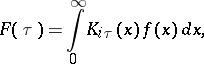where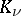is the Macdonald function.

Ifis of bounded variation in a neighbourhood of a point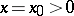and ifthen the following inversion formula holds: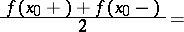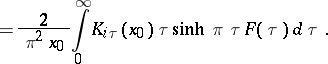Let,, be real-valued functions with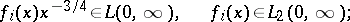and let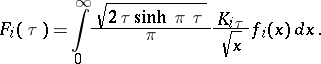Then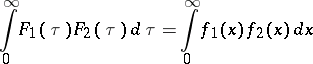(Parseval's identity).

The finite Kontorovich–Lebedev transform has the form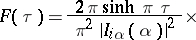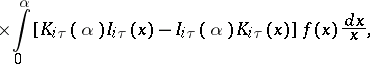, whereis the modified Bessel function (see ).

The study of such transforms was initiated by M.I. Kontorovich and N.N. Lebedev (see , ).